# The Power Of Financial Compounding Explained In 7 Graphs

Jul. 24, 2018 4:08 AM ET2 Likes

This post is inspired by the presentation by Kaushik Punjabi at London Value Investing Club. The content of the post, however, is the view of the author.

We always hear we should start to save for retirement early. We should reinvest our dividends and find products that offer compounding. A slightly higher interest rate can translate into a much higher final value. How true are these? How powerful is compounding interest? It depends on the three factors of 1) time, 2) interest rate, and 3) recurring investment.

### The Three Factors

#### 1. Time

The first factor is time. The simple compound interest formula, with no further investments, is

Amount t = P ( 1 + rn )nt

Where

• P is the principal amount (initial investment),
• r is the annual nominal interest rate,
• n is the number of times interest is compounded per year,
• t is the number of compounding periods.

In its fundamental form, this is a power function y = c x (some constant number raised to a variable exponent and a principal of "1"). If we look at this graph of the fundamental power function, we can see the change in y as x increases. If you use the formula y = c x where c is 3, and x keeps on increasing from 1 to 5, you can see that y increases from 3 to 243.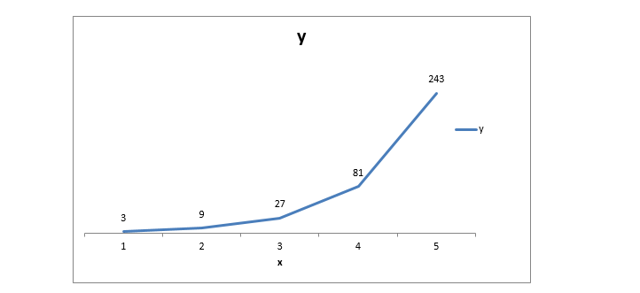In finance, we have much smaller constants than three (three would be a 300% return per period), but the idea holds. The longer an interest rate is applied to an investment, the more "powerful" the interest becomes. It should also be noted that the output for the chart is applied to a principal of 1 (USD, GBP, EUR, whatever). We have much bigger principals and longer timeframes in finance.

A more realistic example in finance:

The chart below represents a £1,500 one-time investment with a constant 5% interest rate. The first one started investing at the beginning of Year 0 and the second at the beginning of Year 10 (i.e., 10 years later). By retirement time (40 years compounding for Year 0, 30 years compounding for Year 10), the earlier investment is worth 63% more.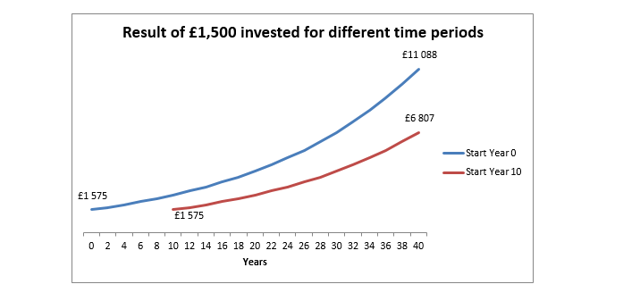#### 2. Interest Rate

The second important factor is the interest rate. As interest rate grows, so does the return over time. In exaggerated numbers, we can see that 33 (27) is much bigger than 23 (8) when compared to initial base values of two and three.

This difference in rates (3-2 = 1 = 100%) rarely occurs in finance, but the principle holds on smaller scales. On short scales, (like the three periods in our example), the difference tends to be small. But on longer timescales, such as 40 periods (forty years of investment), we can see the differences: (1+5%)40 = 700% while (1+8%)40 = 2100%.

The chart below shows the percentage return for a simple, one-time-deposit compounding investment value over forty years. The only variable is the interest rate. As we can see, the return grows exponentially, just like how the time component affects the return. At the end of forty years, 3% returns us 325% while the 10% rate gave us 4500%. For a £1,500 initial deposit, that's £4,875 versus £67,500.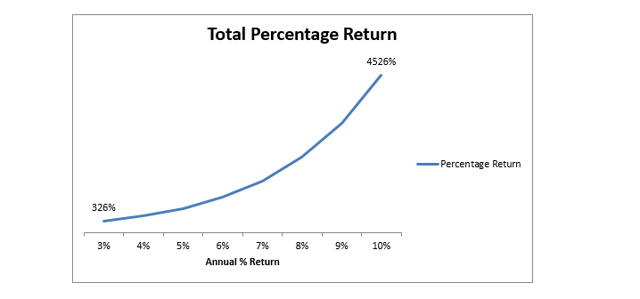#### 3. Recurring Investments

In the above examples, we made simple, one-time deposits then relied entirely on compounding interest to provide returns. But in most investment and savings schemes, we make periodic deposits to increase our principal.

There is no closed-form function for this, so we have to use a recursive function. If we assume the deposit is made at the beginning of the year, so that the interest is applied to the deposit, we get the following:

Amount this period = ( Amount last period + deposit )×( 1 + interest rate )

We must recalculate for every period, as Amount last period changes for every period. When you make deposits, you not only increase the base principal (the amount of pure investment) but you also increase the amount that earns interest.

This is why starting early is important. If you deposit £500 at the beginning of every year and started in Year 10, you would miss out on one interest payment for the Year 9 deposit, two years of interest for the Year 8 deposit, and so on. Your initial deposit would grow by £5,000, but you would also earn interest of about £1,300 on just the deposits over the 10 years.

This chart shows the final value of a 5%, 40-year investment with an initial deposit of £1,500 and varying amounts of yearly deposits.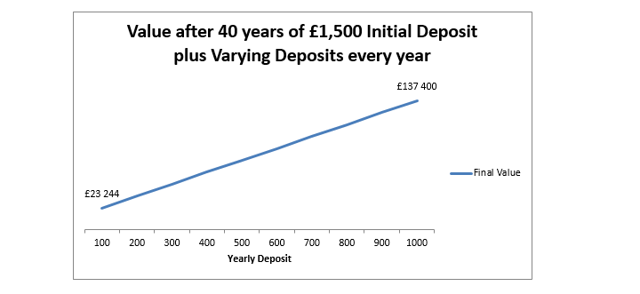We can see changes in the yearly deposit are less important than changes in interest or time, since we have a linear relationship instead of an exponential one. But in absolute numbers, the deposit is still important. £140,000 is much greater than £20,000. By depositing £100 40 times, we end up with an extra £4000 of investment, so we have about £17,500 in interest earned. With £1000 deposited every year, we deposit an extra £40,000, but our total interest earned is much higher at £96,000.

We earned more interest on the more interest produced by the higher deposits. The takeaway? More interest begets more interest (and this is the reason for the saying "the rich get richer").

### What if we don't reinvest our dividends or interest?

Perhaps we want to simply accumulate our interest payments, but we do not reinvest them. If we do this, we will not earn interest on them. That means we have non-compounding interest. How does it compare?

This chart shows an initial investment of £1,500 with no other deposits (so we just completely ignore recurring payments). We have two variables: one is the interest rate (5% vs. 6%), and the other is compounding or not (CIE = compounding interest earned, NCIE = non-compounding interest earned).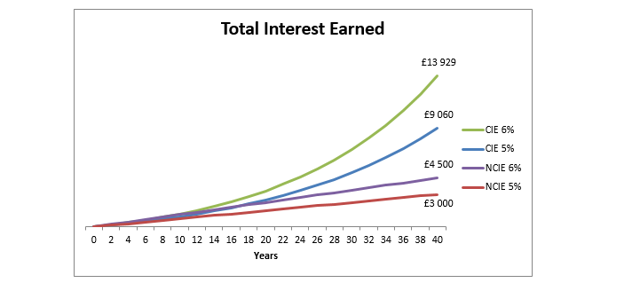The NCIE lines are linear because we simply multiply the number of periods by the interest we generate every year. We make £75 of interest on the £1,500 deposit (for 5%), and that never changes. We just slowly accumulate it, £75 at a time. But with compounding, we can see the first year is £75, but the next year we make £78.75 [(1500+75)×1.05 - 1575], an extra £3.75, which itself contributes to future interest accumulation. This continues to grow over time.

Here is the same chart with compounding for 5% and 6%, but with yearly deposits of £300: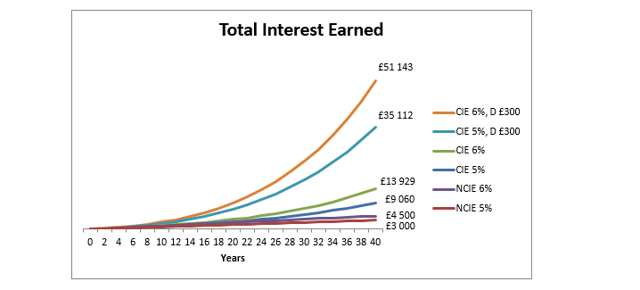And one last chart, showing what would happen if we started an investment scheme with £250 deposits at 5%, but we started it 10 years later.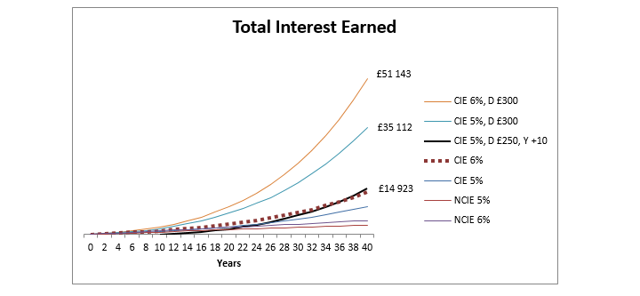The most important thing to note here is that, because the investment is started 10 years later and the interest rate is lower, the investor must deposit £250 every year just to match the 6%, no-deposit line. Over the 30-year-period, that's an extra £7,500 out-of-pocket just because of a late start and an interest rate that is 1% lower.

Moreover, we should also consider that 40 years of £300 deposits will add £12,000 in principal, on top of the original investment and the interest earned.

### Summary

The two main components of compounding interest (time and interest rate) coupled with recurring deposits can make a huge difference in how much your investment grows over time. The return of equities between 2004 and 2017 was 7.7% (Source: Schroders). That means an even more dramatic divergence between non-compounding and compounding plans. And as we can see, if you are leaving money for late retirement, your children, or even your grandchildren, compounding interest only becomes more powerful with time.

One important point to note is that your compounding interest should be above inflation. Keeping assets in very low-interest-yield accounts will not cover inflation, which means the raw amount of money will grow but its buying power will remain stagnant (or, in the worst case scenario, even decrease).

Disclosure: Author and stakeholders of CityFALCON may have vested interest in the financial securities discussed in the article.